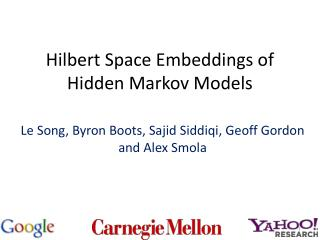DownloadDownload PresentationHilbert Space Embeddings of Hidden Markov Models

# Hilbert Space Embeddings of Hidden Markov Models

Download Presentation## Hilbert Space Embeddings of Hidden Markov Models

- - - - - - - - - - - - - - - - - - - - - - - - - - - E N D - - - - - - - - - - - - - - - - - - - - - - - - - - -
##### Presentation Transcript

1. Hilbert Space Embeddings of Hidden Markov Models Le Song, Byron Boots, SajidSiddiqi, Geoff Gordon and Alex Smola

2. Big Picture Question Dependent variables Hidden variables High dimensional Nonlinear Multimodal High dimensional Nonlinear Multimodal Dependent variables Hidden variables Combine the best of graphical models and kernel methods?

3. Hidden Markov Models (HMMs) Video sequence … … Music • High-dimensional features • Hidden variables • Unsupervised learning

4. Notation Transition Prior Observation … …

5. Previous Work on HMMs • Expectation maximization [Dempster et al. 77]: • Maximum likelihood solution • Local maxima • Curse of dimensionality • Singular value decomposition (SVD) for surrogate hidden states • No local optima • Consistent • Spectral HMMs[Hsu et al. 09, Siddiqi et al. 10],Subspace Identification[Van Overschee and De Moor 96]

6. Predictive Distributions of HMMs • Input output • Variable elimination: Observable Operator [Jaeger 00] =

7. Predictive Distributions of HMMs • Input output • Variable elimination (matrix representation): …

8. Observable representation of HMM • Key observation: need not recover : • where are singular vectors of joint probability of sequence pairs [Hsu et al. 09] Only need to estimate O, Ax and π up to invertible transformation S

9. Observable representation for HMM pairs triplets singletons sequence Thin SVD of C2,1, get principal left singular vectors U

10. Observable representation for HMMs pairs triplets singletons Works only for discrete case

11. Key Objects in Graphical Models • Marginal distributions • Joint distributions • Conditional distributions • Sum rule • Product rule Use kernel representation for distributions, do probabilistic inference in feature space

12. Embedding distributions • Summary statistics for distributions : • Pick a kernel , and generate a different summary statistic Mean Covariance Probability P(y0) expected features

13. Embedding distributions • One-to-one mapping from to for certain kernels (RBF kernel) • Sample average converges to true mean at

14. Embedding joint distributions • Embedding joint distributions using outer-product feature map • is also the covariance operator • Recover discrete probability with delta kernel • Empirical estimate converges at

15. Embedding Conditionals • For each value X=x conditioned on, return the summary statistic for • Some X=x are never observed

16. Embedding conditionals avoid data partition Conditional Embedding Operator

17. Conditional Embedding Operator • Estimation via covariance operators [Song et al. 09] • Gaussian case: covariance matrix instead • Discrete case: joint over marginal • Empirical estimate converges at

18. Sum and Product Rules Total Expectation Conditional Embedding Linearity

19. Hilbert space HMMs pairs triplets singletons

20. Experiment • Video sequence prediction • Slot car sensor measurement prediction • Speech classification • Compare with discrete HMMs learned by EM [Dempster et al. 77], spectral HMM [Sajid et al. 10], and Linear dynamical system approach (LDS) [Sajid et al. 08]

21. Predicting Video Sequences • Sequence of grey scale images as inputs • Latent space dimension 50

22. Predicting Sensor Time-series • Inertial unit: 3D acceleration and orientation • Latent space dimension 20

23. Audio Event Classification • Mel-Frequency CepstralCoefficients features • Varying latent space dimension

24. Summary • Represent distributions in feature spaces, reason using Hilbert space sum and product rules • Extends HMMs nonparametrically to domains with kernels • Kernelizebelief propagation, CRF and general graphical models with hidden variables?